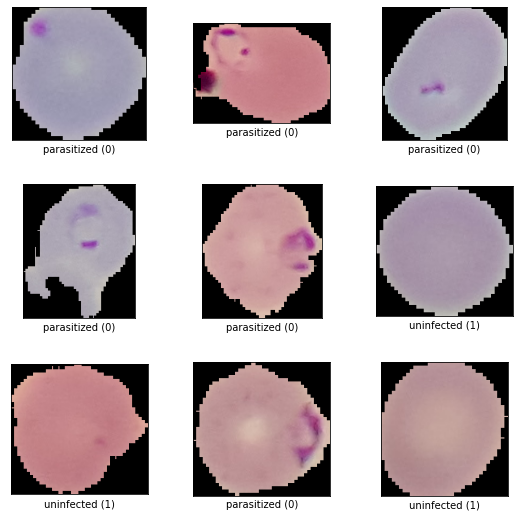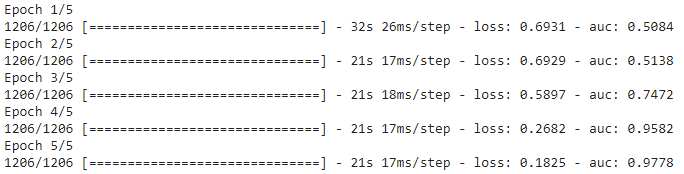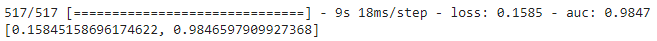# Creating a convolutional network with Tensorflow

## Introduction

They say that a picture is worth a thousand words, but being able to interpret them and extract relevant information from them is worth much more. To carry out this purpose, artificial intelligence provides us with very interesting tools.

In previous blog articles we have seen how to create a neural network, a perceptron, from scratch. However, for more complex problems such as disease detection in images, a simple neural network may not be the best solution. That is why today we introduce convolutional neural networks, a type of image-oriented artificial neural network.

The aim of this article is to carry out a simple demo of how to create a neural network using Tensorflow machine learning library. However, before going into the code, it is advisable to correctly define a set of concepts related to this implementation.

## Basic concepts

Convolutional neural networks are a type of neural network oriented towards the use of images as input or data types that are representable in matrix format. Before going deeper into this article, it is useful to know the concept of a neural network and the simple perceptron. To do so, I recommend reading the articles on our Damavis blog:

After reviewing the basic concepts of the perceptron we can define the vocabulary we will need in this case. First of all, we already know what an artificial neural network is, which we could define as a set of neurons where each one of them represents simple functions and which, when connected together, create a more complex network of connections that allows us to extract patterns from a set of data.

Unlike the aforementioned article, in this case we will not create the convolutional network from scratch but we will use the Tensorflow machine learning library. This library allows us to define the hyperparameters and layers of the network in a simpler way, as well as the training and evaluation of results, among many other functionalities. In other words, a complete view of the typical pipeline in the creation of these artificial intelligence models.

## Our radio’s potentiometers

So, the other two concepts that we need to define before getting into the code, the hyperparameters and the layers, have already appeared. As we have seen in previous articles, the neurons of the network have different types of parameters that define the output of each neuron. We distinguish between two types: parameters and hyperparameters. In reality, the parameters, known as weights, are nothing more than the different factors that properly define the function that each neuron represents. That is, if the neuron represents the function of a line defined by the following formula:

We can observe that the dependent variable ŷ will be determined by two factors or weights, ω0 and ω1 on the independent variable x . The actual value of these weights is derived from training and are thus known as trainable parameters.

On the other hand, we find the hyperparameters that, unlike the other parameters, are defined before the training starts. In addition, they determine the learning process and are the values that, through an iterative process during the search for the best model, will be modified to find the set of hyperparameters that lead to the best performance.

An example of a hyperparameter in any neural network is the learning rate, which defines the variation of the weights in each iteration of the learning process in order to approach the optimal point. In other words, it defines how big each step our model must be during training. In the case of convolutional networks, another common hyperparameter could be the size of the input images or the size of the convolutional filters in each layer.

## Not only heroes wear capes

In this way we introduce the last basic concept: layers and their properties. As we have been able to understand, neural networks of any kind are defined by neurons connected to each other. The connections of these neurons are not anarchic but define a certain hierarchy between them. This organisation allows neurons with similar purposes to be grouped together. Thus, following the architecture of an artificial neural network, a set of neurons will be executed in a certain order. Commonly, in more sophisticated neural networks, these groupings are known as layers. It is the succession and connection between different layers that ultimately defines the architecture of a particular model.

Layers usually have a specific purpose where we can find a multitude of types. Among the most common cases we find: dimensionality reduction layers, such as Average Pooling or Max Pooling layers; neuron disconnection layers to avoid overfitting, such as Dropout layers; or convolutional layers, among many others.

As far as convolutional layers are concerned, a special mention should be made, as it is not by chance that they give their own name to the networks that we will implement in this booklet. A convolution can be defined mathematically with the following formula:

If we pay attention to it, we can observe that this definition implies two functions: f and g along a domain t . Convolution can be understood as the product of two functions whose result defines the magnitude of both when they overlap. In other words, one function is slid over the other by integrating the product of both and the result defines a new function.

The understanding of its use in neural networks involving images is immediate if the matrix of an image is understood as one of the functions. On the other hand, the second function that will slide along the image will be a filter. Filters are nothing more than matrices, with a dimension smaller than that of the original image, that represent a particular pattern. In the earliest layers of the network these filters will define simple patterns such as straight lines or edges within the image. While in the deeper layers these filters will be more complex, thus defining much more elaborate patterns on the image.

Thus, sliding the filter over the image as a convolution of these two functions will result in a new image, a new function, where pixels that fit the pattern represented by the filter will be highlighted.

Once the basics of convolutional neural networks are known, we can move on to their implementation in code using Tensorflow.

## Implementation

The most relevant steps of the Python development for the creation of the convolutional network are described below. The complete implementation can be found at the following Jupyter notebook: damavis/blog-posts-code/notebooks/cnn_demo.ipynb

## Data: Malaria Dataset

The dataset used belongs to the Tensorflow Datasets data catalogue. This dataset has been used since the aim of this workbook is not to carry out preprocessing on it. In this way we obtain an image dataset that meets our desired requirements, clean and focused on the problem of image classification.

In the Malaria dataset, images of fine blood smear slides of segmented cells are represented. We will have two classes: parasitized cells and uninfected cells.

### Data at a glance

If we look at the data we are going to use, we can see in the following image an example of the different classes of our dataset. On the one hand we have images of parasitised cells and on the other of uninfected cells. In our case, the dataset consists of 19,291 training images and 8,267 test images.

``````(train_ds, test_ds), info = tfds.load("malaria", split=['train[:70%]', 'train[70%:]'], shuffle_files=True, with_info=True, as_supervised=True)
``````

Using the function `tfds.load()` we load the Malaria dataset from the Tensorflow catalogue.Figure 1. Examples of the images in the dataset.

### Dataset pre-processing

Even though the data is already pre-processed and clean, we have to perform a slight pre-processing in order to be able to load the data into our model correctly. In this case the most relevant transformation is to resize the image to a common size for the whole set that is not too large. Although it may seem counter-intuitive, a larger number of pixels does not always result in better model performance. In addition, the larger the image dimensions, the more pixel sets the network has to process and therefore the more time consuming it is.

For the realisation of this demo a size of 28×28 pixels is sufficient.

``````def preprocessing(img, label):
# Transformamos el tipo de la imagen
image_converted = tf.image.convert_image_dtype(img, tf.float32)
# Agregamos padding a la imagen
image_resized = tf.image.resize(image_converted, (IMG_HEIGHT, IMG_WIDTH))

return image_resized, label

processed_train_ds = train_ds.cache().map(preprocessing).batch(BATCH_SIZE)
processed_test_ds = test_ds.cache().map(preprocessing).batch(BATCH_SIZE)``````

Thanks to the `preprocessing()` function we can map the necessary transformations for each of the images in our dataset. In this way, we get two new dataset partitions where all images have the same properties.

## Model creation

At this point we can carry out the construction of our convolutional network. Using Tensorflow, defining the sequential organisation of layers is very simple. In this case we will use the layers mentioned in the basic concepts section of this article.

``````def build_model():
model = tf.keras.Sequential([
tf.keras.Input(shape=(IMG_HEIGHT, IMG_WIDTH, CHANNELS)),
tf.keras.layers.Conv2D(filters=16,
kernel_size=3,
activation='relu',

tf.keras.layers.MaxPool2D(),

tf.keras.layers.Conv2D(filters=32,
kernel_size=3,
activation='relu',

tf.keras.layers.MaxPool2D(),
tf.keras.layers.Dropout(0.2),

tf.keras.layers.Flatten(),

tf.keras.layers.Dense(8, activation='relu'),
tf.keras.layers.Dropout(0.2),

tf.keras.layers.Dense(1, activation='sigmoid')
])

return model``````

The network architecture consists of a first input layer, followed by convolution layers, max pooling, dropout and dense layers. The convolution layers will extract the characteristic patterns of each image. The max pooling layers will reduce the image dimensions by a process of selecting the highest value in a set of pixels. Finally, dense layers are nothing more than sets of interconnected neurons, also known as fully connected layers. Dropout layers will mitigate model overfitting by randomly disconnecting some connections between neurons.

``````CNN_model.compile(
loss=tf.keras.losses.binary_crossentropy,
metrics=[tf.keras.metrics.AUC(name='auc')]
)
``````

In Tensorflow, once the model has been created, it must be compiled due to its internal management of the tensors. It is in this compile() function where we define some of the hyperparameters such as the learning rate, the loss function or the metric to evaluate the model, in this case the AUC.

## Training

In this section we have considered carrying out a short training of only 5 iterations or epochs. With only 5 iterations we can already observe that the loss of the model decreases after each one of them and that our evaluation metric increases subtly. The metric used was the AUC, area under the curve, which defines a type of ROC curve that relates the rate of true positives to the rate of false positives. The higher the value, the better the performance of our model. It is a commonly used metric in disease detection.

``````history = CNN_model.fit(
processed_train_ds,
epochs=EPOCHS
)``````Figure 2. Output of the execution of the fit() function.

## Evaluation

``CNN_model.evaluate(processed_test_ds)``Figure 3. Output from the execution of the evaluate() function.

In the evaluation of the model it is observed that its performance in the test subset is optimal reaching 98.47% AUC.

## That’ s all folks!

This is everything… or almost everything. In this publication we have seen how easy it is to create an artificial intelligence model capable of classifying images. However, we started from very specific conditions, as we did not have to go through many of the usual pipeline steps.

It was not necessary to do a very exhaustive pre-processing of the data, nor to carry out any iterative process to get to know the architecture that would lead us to a model with high performance, nor did we need to serialise and deploy the model in production so that it could be consumed by a client. These are all important steps in the pipeline of a machine learning project and, as in this publication, require time.

In the next publications we will address each of these phases in depth. And so we will be able to get a complete overview of the whole process.

If you found this post interesting, we encourage you to share this article on social media. Don’t forget to mention us to let us know what you think (@DamavisStudio), see you networks!